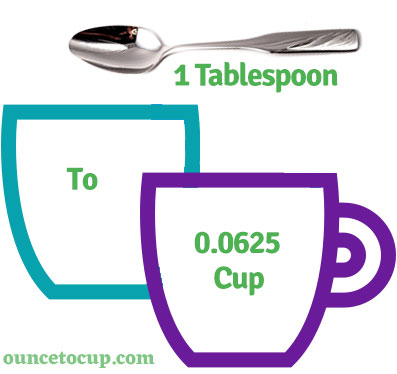# 3 Tablespoons to Cups (3 tbsp to c conversion)

Are you cooking your favorite dish? The detailed chart in the recipe includes the calculation of the 3 tablespoon to cup conversion.  Don't worry; use this calculator to determine how many 3 tablespoon equals cups in a minute.  This 3 tbsp to c converter gives an exact measurement for any recipe you prepare.

Tablespoon Value:

tbsp

Cup Value:

c

3 Tablespoon = 0.1875 Cup
(3 tbsp = 0.1875 c)

Try our auto 3 Tablespoon to Cup Calculator (Without Convert Button), Just change the first field value and you got final value.## How many cup is a 3 tbsp?

We know that the mass value of 3 tbsp is equal to 0.1875 c. If you want to convert 3 tbsp to an equal number of cup, just divide the volume value by 16. Hence, 3 tablespoon is equal to 0.1875 cup.

The Answer is: 3 US Tablespoons = 0.1875 US Cup

3 tbsp = 0.1875 cup

Many of them try to search or find an answer for what is 3 tablespoons in c? So, we’ll start with 3 tbsp to c conversion to know how big is 3 tbsp.

## How To Calculate 3 tbsp to cup?

To calculate 3 tablespoons to an equal number of cup, simply follow the steps below.

Tablespoons to Cups formula is:

Cup = Tablespoon / 16

Assume that we are finding out how many cup were found in 3 tbsp, divide by 16 to get the result.

Applying to Formula: cup = 3 tbsp / 16 = 0.1875 cup.

## How To Convert 3 tbsp to cup?

• To convert 3 tablespoons to cup,
• Simply divide the tablespoon value by 16.
• Applying to the formula, cup = 3 tablespoons / 16 [3/16].
• Hence, 3 tablespoons is equal to 0.1875 cup.
Tablespoons [tbsp]Cups [c]
1 tbps0.0625 cup
2 tbps0.125 cup
3 tbps0.1875 cup
4 tbps0.25 cup
5 tbps0.3125 cup
6 tbps0.375 cup
7 tbps0.4375 cup
8 tbps0.5 cup
9 tbps0.5625 cup
10 tbps0.625 cup
11 tbps0.6875 cup
12 tbps0.75 cup
13 tbps0.8125 cup
14 tbps0.875 cup
15 tbps0.9375 cup
16 tbps1 cup

## Reverse Calculation: How many tablespoons are in 3 cups?

• To convert 3 cups to tbsp,
• Simply multiply the 3 cup by 16.
• Then, applying the formula, tbsp = 3 cup * 16 [3x16 = 48].
• Hence, 3 cup is equal to 48 tablespoon.

### Related Converter:

Formula: tablespoon to cup

cup = tablespoon / 16

Applying to Formula,

c = 3/16 = 0.1875

3 tablespoon = 0.1875 cup